085-1263404

### My Journal

All things Mathematical
19 Sep 2018
##### Question 1

A wheel rolls uniformly on the ground, without sliding, its centre describing a straight line with uniform velocity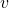. Show that at any instant every point on the rim is turning about the point of contact of the wheel with the ground with an angular velocity equal to that of the wheel about its centre.

##### Question 2

A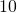ton lorry attains a speed of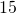miles per hour from rest in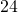seconds during which time it travels a distance of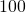yards. If the average resistance to motion over this distance is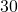lbs. per ton-find the average Horse Power required.

##### Question 3

If the hodograph of the path of a moving point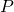be drawn, then the velocity of the corresponding point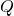in the hodograph represents in magnitude and direction the acceleration ofin its path.

Prove this theorem and use it to determine the magnitude and direction of the acceleration of a point moving in a circle of radius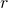with a uniform speed.

##### Question 4

The bob of a conical pendulum is moving in a horizontal circle with uniform velocity. With the aid of a clear diagram indicate the forces acting on it at any instant and analyse the motion.

If the bob makes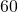revolutions per minute, what is the vertical distance of the circle of rotation below the point of suspension of the pendulum?

##### Question 5

A machine part weighinglbs. has a simple harmonic motion in a straight line. The distance between the end points of its travel is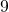inches and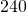complete to-and-fro movements are made per minute. Calculate :-

(a) the acceleration and the accelerating force when it is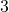inches from its mid-position;

(b) its maximum velocity;

(c) the maximum accelerating force.

##### Question 6

Neglecting frictional resistance, calculate the least velocity with which a truck must enter the lowest point of a vertical circular track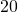feet in diameter in order to just “loop the loop.”

Find also the velocity of the truck and the reaction of the track when half way up.

Weight of truck =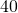lbs.

##### Question 7

Two long pendulums of equal length are suspended so that their bobs just touch when at rest. The masses of the bobs are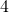lbs. and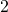lbs. respectively. They are pulled apart in opposite directions-the horizontal displacements from the rest positions being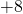inches andinches respectively-and released simultaneously. In impact they join together and move as one body. The compound mass is found to have a maximum horizontal displacement of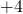inches from the mean positions.

On the assumption that the horizontal displacements represent to some scale the velocities of the masses at their mean positions,  show that these results verify the Principle of Conservation of Momentum.

Justify this assumption.

##### Question 8

Over a smooth pulley is passed a light string supporting at one end a mas oflbs. and at the other a pulley of masslb. A string with masses oflbs. andlbs. attached to its ends passes over the second pulley.

Find the acceleration of each of the moving bodies and of the centre of gravity of the moving system.

##### Question 9

A box weighing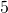lbs. placed on a plank just slides down when the plank is tilted at an angle of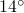to the horizontal.

The plank is then fixed at an incline of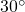to the horizontal and a weight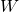lbs. is placed in the box. Find an equation showing the effortlbs. acting parallel to the incline which will drag the box and weight up the incline at a slow uniform speed.

Write down expressions for the Mechanical Advantage and Efficiency of the arrangement.

##### Question 10

Two poles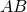and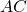jointed together at their tops and making an angle of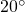with each other are used as shear legs. The ends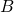and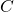rest on the level ground an the plane of triangle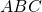is inclined at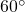to the horizontal. A back stay joined at the top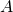and to a point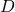on the ground behind the legs is placed symmetrically with respect to them and makes angle ofwith the plane of the legs.

Find the forces in the legs and back stay when a load oftons is hung from.

##### Citation:

State Examinations Commission (2018). State Examination Commission. Accessed at: https://www.examinations.ie/?l=en&mc=au&sc=ru

Malone, D and Murray, H. (2016). Archive of Maths State Exams Papers. Accessed at: http://archive.maths.nuim.ie/staff/dmalone/StateExamPapers/

##### Licence:

“Contains Irish Public Sector Information licensed under a Creative Commons Attribution 4.0 International (CC BY 4.0) licence”.

The EU Directive 2003/98/EC on the re-use of public sector information, its amendment EU Directive 2013/37/EC, its transposed Irish Statutory Instruments S.I. No. 279/2005, S.I No. 103/2008, and S.I. No. 525/2015, and related Circulars issued by the Department of Finance (Circular 32/05), and Department of Public Expenditure and Reform (Circular 16/15 and Circular 12/16).

Note. Circular 12/2016: Licence for Re-Use of Public Sector Information adopts CC-BY as the standard PSI licence, and notes that the open standard licence identified in this Circular supersedes PSI General Licence No: 2005/08/01.